# 7 Coordinate Systems, Transformations and Units

## 7.1 Introduction

For all media, the SVG canvas describes "the space where the SVG content is rendered." The canvas is infinite for each dimension of the space, but rendering occurs relative to a finite rectangular region of the canvas. This finite rectangular region is called the SVG viewport. For visual media [CSS2-VISUAL], the SVG viewport is the viewing area where the user sees the SVG content.

The size of the SVG viewport (i.e., its width and height) is determined by a negotiation process (see Establishing the size of the initial viewport) between the SVG document fragment and its parent (real or implicit). Once that negotiation process is completed, the SVG user agent is provided the following information:

• an integer value that represents the width in "pixels" of the viewport
• an integer value that represents the height in "pixels" of the viewport
• (highly desirable but not required) a real number value that indicates how many millimeters a "pixel" represents

Using the above information, the SVG user agent determines the viewport, an initial viewport coordinate system and an initial user coordinate system such that the two coordinates systems are identical. Both coordinates systems are established such that the origin matches the origin of the viewport, and one unit in the initial coordinate system equals one "pixel" in the viewport. (See Initial coordinate system.) The viewport coordinate system is also called viewport space and and the user coordinate system is also called user space.

Lengths in SVG can be specified as:

• (if no unit designator is provided) values in user space -- for example, "15"
• (if a CSS unit specifier is provided) a length in CSS units -- for example, "15mm"

The supported CSS length unit specifiers are: em, ex, px, pt, pc, cm, mm, in, and percentages.

A new user space (i.e., a new current coordinate system) can be established at any place within an SVG document fragment by specifying transformations in the form of transformation matrices or simple transformation operations such as rotation, skewing, scaling and translation. Establishing new user spaces via coordinate system transformations are fundamental operations to 2D graphics and represent the usual method of controlling the size, position, rotation and skew of graphic objects.

New viewports also can be established. By establishing a new viewport, you can redefine the meaning of some of the various CSS unit specifiers (px, pt, pc, cm, mm, in, and percentages) and provide a new reference rectangle for "fitting" a graphic into a particular rectangular area. ("Fit" means that a given graphic is transformed in such a way that its bounding box in user space aligns exactly with the edges of a given viewport.)

## 7.2 The initial viewport

The SVG user agent negotiates with its parent user agent using any CSS positioning parameters on the outermost 'svg' element and the width= and height= XML attributes that are required on the 'svg' element to determine the viewport into which the SVG user agent can render the document. In the negotiation process, if the parent document uses CSS positioning and the outermost 'svg' element contains CSS positioning properties [CSS2-POSN] which are sufficient to establish the width of the viewport, then the CSS positioning properties establish the viewport's width; otherwise, the width= attribute on the outermost 'svg' element establishes the viewport's width. Similarly, if the parent document uses CSS positioning and the outermost 'svg' element contains CSS positioning properties [CSS2-POSN] which are sufficient to establish the height of the viewport, then the CSS positioning properties establish the viewport's height; otherwise, the height= attribute on the outermost 'svg' element establishes the viewport's height.

If the width= or height= attributes on the outermost 'svg' element are in user units (i.e., no unit specifier has been provided), then the value is assumed to be equivalent to the same number of CSS "px" units.

In the following example, an SVG graphic is embedded within a parent XML document which is formatted using CSS layout rules. Since CSS positioning properties are not provided on the outermost 'svg' element, the width="100px" and height="200px" attributes determine the size of the initial viewport:

```<?xml version="1.0" standalone="yes"?>
<parent xmlns="http://some.url">

<!-- SVG graphic -->
<svg xmlns='http://www.w3.org/2000/svg-20000303-stylable'
width="100px" height="200px">
<path d="M100,100 Q200,400,300,100"/>
<!-- rest of SVG graphic would go here -->
</svg>

</parent>
```

Download this example

The initial clipping path for the SVG document fragment is established according to the rules described in The initial clipping path.

## 7.3 The initial coordinate system

For the outermost 'svg' element, the SVG user agent determines an initial viewport coordinate system and an initial user coordinate system such that the two coordinates systems are identical. The origin of both coordinate systems is at the origin of the viewport, and one unit in the initial coordinate system equals one "pixel" in the viewport. In most cases, such as stand-alone SVG documents or SVG document fragments embedded within XML parent documents where the parent's layout is determined by CSS [CSS2] or XSL [XSL], the initial viewport coordinate system (and therefore the initial user coordinate system) has its origin at the top/left of the viewport, with the positive X axis pointing towards the right, the positive Y axis pointing down, and text rendered with an "upright" orientation, which means glyphs are oriented such that Roman characters and full-size ideographic characters for Asian scripts have the top edge of the corresponding glyphs oriented upwards and the right edge of the corresponding glyphs oriented to the right.

Example InitialCoords below shows that the initial coordinate system has the origin at the top/left with the X axis pointing to the right and the Y axis pointing down. The initial user coordinate system has one user unit equal to the parent (implicit or explicit) user agent's "pixel".

```<?xml version="1.0" standalone="no"?>
<!DOCTYPE svg PUBLIC "-//W3C//DTD SVG 20000303 Stylable//EN"
"http://www.w3.org/TR/2000/03/WD-SVG-20000303/DTD/svg-20000303-stylable.dtd">
<svg width="300px" height="100px">
<desc>Example InitialCoords - SVG's initial coordinate system</desc>

<g style="fill:none; stroke:black; stroke-width:3">
<line x1="0" y1="1.5" x2="300" y2="1.5" />
<line x1="1.5" y1="0" x2="1.5" y2="100" />
</g>
<g style="fill:red; stroke:none">
<rect x="0" y="0" width="3" height="3" />
<rect x="297" y="0" width="3" height="3" />
<rect x="0" y="97" width="3" height="3" />
</g>
<g style="font-size:14 font-family:Verdana">
<text x="10" y="20">(0,0)</text>
<text x="240" y="20">(300,0)</text>
<text x="10" y="90">(0,100)</text>
</g>
</svg>
```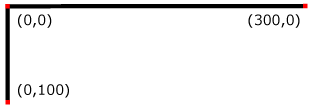## 7.4 Coordinate system transformations

A new user space (i.e., a new current coordinate system) can be established by specifying transformations in the form of a transform attribute on a container element or graphics element. The transform attribute transforms all user space coordinates and lengths on the given element and all of its ancestors. Transformations can be nested, in which case the effect of the transformations are cumulative.

The following demonstrates simple transformations:

Example OrigCoordSys below shows a document without transformations. The text string is specified in the initial coordinate system.

```<?xml version="1.0" standalone="no"?>
<!DOCTYPE svg PUBLIC "-//W3C//DTD SVG 20000303 Stylable//EN"
"http://www.w3.org/TR/2000/03/WD-SVG-20000303/DTD/svg-20000303-stylable.dtd">
<svg width="400px" height="150px">
<desc>Example OrigCoordSys - Simple transformations: original picture</desc>
<g style="fill:none; stroke:black; stroke-width:3">
<!-- Draw the axes of the original coordinate system -->
<line x1="0" y1="1.5" x2="400" y2="1.5" />
<line x1="1.5" y1="0" x2="1.5" y2="150" />
</g>
<g>
<text x="30" y="30" style="font-size:20 font-family:Verdana">
ABC (orig coord system)
</text>
</g>
</svg>
```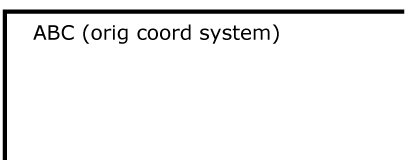Example NewCoordSys establishes a new user coordinate system by specifying transform="translate(50,50)" on the third 'g' element below. The new user coordinate system has its origin at location (50,50) in the original coordinate system. The result of this transformation is that the coordinate (30,30) in the new user coordinate system gets mapped to coordinate (80,80) in the original coordinate system (i.e., the coordinates have been translated by 50 units in X and 50 units in Y).

```<?xml version="1.0" standalone="no"?>
<!DOCTYPE svg PUBLIC "-//W3C//DTD SVG 20000303 Stylable//EN"
"http://www.w3.org/TR/2000/03/WD-SVG-20000303/DTD/svg-20000303-stylable.dtd">
<svg width="400px" height="150px">
<desc>Example NewCoordSys - New user coordinate system</desc>
<g style="fill:none; stroke:black; stroke-width:3">
<!-- Draw the axes of the original coordinate system -->
<line x1="0" y1="1.5" x2="400" y2="1.5" />
<line x1="1.5" y1="0" x2="1.5" y2="150" />
</g>
<g>
<text x="30" y="30" style="font-size:20 font-family:Verdana">
ABC (orig coord system)
</text>
</g>
<!-- Establish a new coordinate system, which is
shifted (i.e., translated) from the initial coordinate
system by 50 user units along each axis. -->
<g transform="translate(50,50)">
<g style="fill:none; stroke:red; stroke-width:3">
<!-- Draw lines of length 50 user units along
the axes of the new coordinate system -->
<line x1="0" y1="0" x2="50" y2="0" style="stroke:red"/>
<line x1="0" y1="0" x2="0" y2="50" />
</g>
<text x="30" y="30" style="font-size:20 font-family:Verdana">
ABC (translated coord system)
</text>
</g>
</svg>
```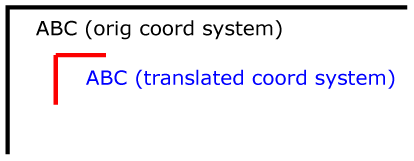Example RotateScale illustrates simple rotate and scale transformations. The example defines two new coordinate systems:

• one which is the result of a translation by 50 units in X and 30 units in Y, followed by a rotation of 30 degrees
• another which is the result of a translation by 200 units in X and 40 units in Y, followed by a scale transformation of 1.5.
```<?xml version="1.0" standalone="no"?>
<!DOCTYPE svg PUBLIC "-//W3C//DTD SVG 20000303 Stylable//EN"
"http://www.w3.org/TR/2000/03/WD-SVG-20000303/DTD/svg-20000303-stylable.dtd">
<svg width="400px" height="120px">
<desc>Example RotateScale - Rotate and scale transforms</desc>
<g style="fill:none; stroke:black; stroke-width:3">
<!-- Draw the axes of the original coordinate system -->
<line x1="0" y1="1.5" x2="400" y2="1.5" />
<line x1="1.5" y1="0" x2="1.5" y2="120" />
</g>
<!-- Establish a new coordinate system whose origin is at (50,30)
in the initial coord. system and which is rotated by 30 degrees. -->
<g transform="translate(50,30)">
<g transform="rotate(30)">
<g style="fill:none; stroke:red; stroke-width:3">
<line x1="0" y1="0" x2="50" y2="0" />
<line x1="0" y1="0" x2="0" y2="50" />
</g>
<text x="0" y="0" style="font-size:20; font-family:Verdana; fill:blue">
ABC (rotate)
</text>
</g>
</g>
<!-- Establish a new coordinate system whose origin is at (200,40)
in the initial coord. system and which is scaled by 1.5. -->
<g transform="translate(200,40)">
<g transform="scale(1.5)">
<g style="fill:none; stroke:red; stroke-width:3">
<line x1="0" y1="0" x2="50" y2="0" />
<line x1="0" y1="0" x2="0" y2="50" />
</g>
<text x="0" y="0" style="font-size:20; font-family:Verdana; fill:blue">
ABC (scale)
</text>
</g>
</g>
</svg>
```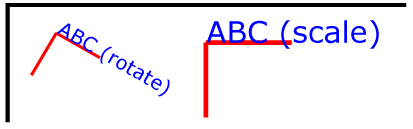Example Skew defines two coordinate systems which are skewed relative to the origin coordinate system.

```<?xml version="1.0" standalone="no"?>
<!DOCTYPE svg PUBLIC "-//W3C//DTD SVG 20000303 Stylable//EN"
"http://www.w3.org/TR/2000/03/WD-SVG-20000303/DTD/svg-20000303-stylable.dtd">
<svg width="400px" height="120px">
<desc>Example Skew - Show effects of skewX and skewY</desc>
<g style="fill:none; stroke:black; stroke-width:3">
<!-- Draw the axes of the original coordinate system -->
<line x1="0" y1="1.5" x2="400" y2="1.5" />
<line x1="1.5" y1="0" x2="1.5" y2="120" />
</g>
<!-- Establish a new coordinate system whose origin is at (30,30)
in the initial coord. system and which is skewed in X by 30 degrees. -->
<g transform="translate(30,30)">
<g transform="skewX(30)">
<g style="fill:none; stroke:red; stroke-width:3">
<line x1="0" y1="0" x2="50" y2="0" />
<line x1="0" y1="0" x2="0" y2="50" />
</g>
<text x="0" y="0" style="font-size:20; font-family:Verdana; fill:blue">
ABC (skewX)
</text>
</g>
</g>
<!-- Establish a new coordinate system whose origin is at (200,30)
in the initial coord. system and which is skewed in Y by 30 degrees. -->
<g transform="translate(200,30)">
<g transform="skewY(30)">
<g style="fill:none; stroke:red; stroke-width:3">
<line x1="0" y1="0" x2="50" y2="0" />
<line x1="0" y1="0" x2="0" y2="50" />
</g>
<text x="0" y="0" style="font-size:20; font-family:Verdana; fill:blue">
ABC (skewY)
</text>
</g>
</g>
</svg>
```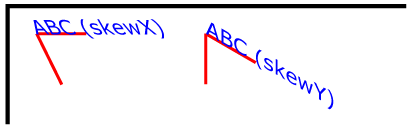Mathematically, all transformations can be represented as 3x3 transformation matrices of the following form: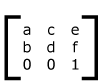Since only six values are used in the above 3x3 matrix, a transformation matrix is also expressed as a vector: [a b c d e f].

Transformations map coordinates and lengths from a new coordinate system into a previous coordinate system: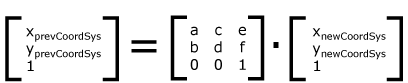Simple transformations are represented in matrix form as follows:

• Translation is equivalent to the matrixor [1 0 0 1 tx ty], where tx and ty are the distances to translate coordinates in X and Y, respectively.

• Scaling is equivalent to the matrix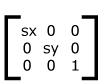or [sx 0 0 sy 0 0]. One unit in the X and Y directions in the new coordinate system equals sx and sy units in the previous coordinate system, respectively.

• Rotation is equivalent to the matrixor [cos(a) sin(a) -sin(a) cos(a) 0 0], which has the effect of rotating the coordinate system axes by angle a.

• A skew transformation along the X axis is equivalent to the matrixor [1 0 tan(a) 1 0 0], which has the effect of skewing X coordinates by angle a.

• A skew transformation along the Y axis is equivalent to the matrix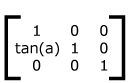or [1 tan(a) 0 1 0 0], which has the effect of skewing Y coordinates by angle a.

## 7.5 Nested transformations

Transformations can be nested to any level. The effect of nested transformations is to post-multiply (i.e., concatenate) the subsequent transformation matrices onto previously defined transformations:For each given element, the accumulation of all transformations that have been defined on the given element and all of its ancestors up to and including the element which established the current viewport (usually, the 'svg' element which is the most immediate ancestor to the given element) is called the current transformation matrix or CTM. The CTM thus represents the mapping of current user coordinates to viewport coordinates: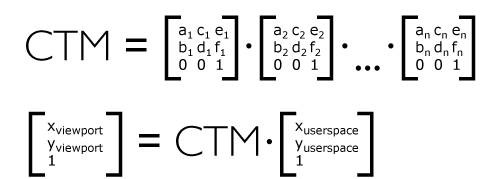Example Nested illustrates nested transformations.

```<?xml version="1.0" standalone="no"?>
<!DOCTYPE svg PUBLIC "-//W3C//DTD SVG 20000303 Stylable//EN"
"http://www.w3.org/TR/2000/03/WD-SVG-20000303/DTD/svg-20000303-stylable.dtd">
<svg width="400px" height="150px">
<desc>Example Nested - Nested transformations</desc>
<g style="fill:none; stroke:black; stroke-width:3">
<!-- Draw the axes of the original coordinate system -->
<line x1="0" y1="1.5" x2="400" y2="1.5" />
<line x1="1.5" y1="0" x2="1.5" y2="150" />
</g>
<!-- First, a translate -->
<g transform="translate(50.90)">
<g style="fill:none; stroke:red; stroke-width:3">
<line x1="0" y1="0" x2="50" y2="0" />
<line x1="0" y1="0" x2="0" y2="50" />
</g>
<text x="0" y="0" style="font-size:16; font-family:Verdana">
....Translate(1)
</text>
<!-- Second, a rotate -->
<g transform="rotate(-45)">
<g style="fill:none; stroke:green; stroke-width:3">
<line x1="0" y1="0" x2="50" y2="0" />
<line x1="0" y1="0" x2="0" y2="50" />
</g>
<text x="0" y="0" style="font-size:16; font-family:Verdana">
....Rotate(2)
</text>
<!-- Third, another translate -->
<g transform="translate(130,160)">
<g style="fill:none; stroke:blue; stroke-width:3">
<line x1="0" y1="0" x2="50" y2="0" />
<line x1="0" y1="0" x2="0" y2="50" />
</g>
<text x="0" y="0" style="font-size:16; font-family:Verdana">
....Translate(3)
</text>
</g>
</g>
</g>
</svg>
```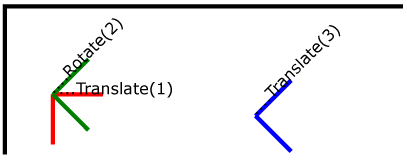In the example above, the CTM within the the third nested transformation (i.e,, the transform="translate(130,160)") consists of the concatenation of the three transformations, as follows: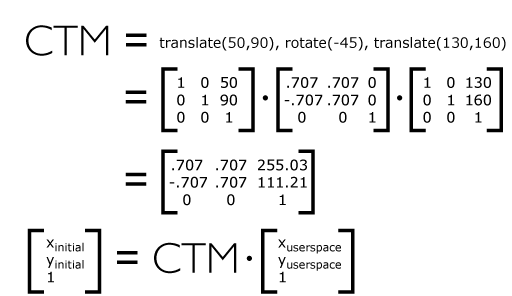## 7.6 The transform attribute

The value of the transform attribute is a <transform-list>, which is defined as a list of transform definitions, which are applied in the order provided. The individual transform definitions are separated by whitespace and/or a comma. The available types of transform definitions include:

• matrix(<a> <b> <c> <d> <e> <f>), which specifies a transformation in the form of transformation matrix of six values. matrix(a,b,c,d,e,f) is equivalent to applying the transformation matrix [a b c d e f]. The e and f values can be specified with CSS unit specifiers.

• translate(<tx> [<ty>]), which specifies a translation by tx and ty. tx and ty values can be specified with CSS unit specifiers.

• scale(<sx> [<sy>]), which specifies a scale operation by sx and sy. If <sy> is not provided, it is assumed to be equal to <sx>.

• rotate(<rotate-angle>), which specifies a rotation by <rotate-angle> about the origin of the current user coordinate system.

• skewX(<skew-angle>), which specifies a skew transformation along the X axis.

• skewY(<skew-angle>), which specifies a skew transformation along the Y axis.

All numeric values are real numbers. All angle values are expressed according to the rules for basic data type <angle>.

If a list of transforms is provided, then the net effect is as if each transform had been specified separately in the order provided. For example,

```<g transform="translate(-10,-20) scale(2) rotate(45) translate(5,10)">
<!-- graphics elements go here -->
</g>
```

is functionally equivalent to:

```<g transform="translate(-10,-20)">
<g transform="scale(2)">
<g transform="rotate(45)">
<g transform="translate(5,10)">
<!-- graphics elements go here -->
</g>
</g>
</g>
</g>
```

The transform attribute is applied to an element before processing any other coordinate or length values supplied for that element. In the element

```<rect x="10" y="10" width="20" height="20" transform="scale(2)"/>
```

the x, y, width and height values are processed after the current coordinate system has been scaled uniformly by a factor of 2 by the transform attribute. Attributes x, y, width and height (and any other attributes or properties) are treated as values in the new user coordinate system, not the previous user coordinate system. Thus, the above 'rect' element is functionally equivalent to:

```<g transform="scale(2)">
<rect x="10" y="10" width="20" height="20"/>
</g>
```

The following is the BNF for values for the transform attribute. The following notation is used:

• *: 0 or more
• +: 1 or more
• ?: 0 or 1
• (): grouping
• |: separates alternatives
• double quotes surround literals
```transform-list:
wsp* transforms? wsp*

transforms:
transform
| transform comma-wsp+ transforms

transform:
matrix
| translate
| scale
| rotate
| skewX
| skewY

matrix:
"matrix" wsp* "(" wsp*
number comma-wsp
number comma-wsp
number comma-wsp
number comma-wsp
length comma-wsp
length wsp* ")"

translate:
"translate" wsp* "(" wsp* length ( comma-wsp length )? wsp* ")"

scale:
"scale" wsp* "(" wsp* length ( comma-wsp number )? wsp* ")"

rotate:
"rotate" wsp* "(" wsp* number wsp* ")"

skewX:
"skewX" wsp* "(" wsp* number wsp* ")"

skewY:
"skewY" wsp* "(" wsp* number wsp* ")"

length:
number unit-specifier?

number:
sign? integer-constant
| sign? floating-point-constant

comma-wsp:
(wsp+ comma? wsp*) | (comma wsp*)

comma:
","

integer-constant:
digit-sequence

floating-point-constant:
fractional-constant exponent?
| digit-sequence exponent

fractional-constant:
digit-sequence? "." digit-sequence
| digit-sequence "."

exponent:
( "e" | "E" ) sign? digit-sequence

sign:
"+" | "-"

digit-sequence:
digit
| digit digit-sequence

digit:
"0" | "1" | "2" | "3" | "4" | "5" | "6" | "7" | "8" | "9"

unit-specifier:
"em"| "ex" | "px" | "pt" | "pc" | "cm" | "mm" | "in" | "%"

wsp:
(#x20 | #x9 | #xD | #xA)
```

For the transform attribute:

Animatable: yes.

See the 'animateTransform' element for information on animating transformations.

## 7.7 The viewBox attribute

It is often desirable to specify that a given set of graphics stretch to fit a particular container element. The viewBox attribute provides this capability.

All elements that establish a new viewport (see elements that establish viewports) have attribute viewBox. The value of the viewBox attribute is a list of four numbers <min-x>, <min-y>, <width> and <height> which specify a rectangle in user space which should be mapped to the bounds of the viewport established by the given element, taking into account attribute preserveAspectRatio. If specified, an additional transformation is applied to all descendants of the given element to achieve the specified effect.

Example ViewBox illustrates the use of the viewBox attribute on the outermost 'svg' element to specify that the SVG content should stretch to fit bounds of the viewport.

```<?xml version="1.0" standalone="no"?>
<!DOCTYPE svg PUBLIC "-//W3C//DTD SVG 20000303 Stylable//EN"
"http://www.w3.org/TR/2000/03/WD-SVG-20000303/DTD/svg-20000303-stylable.dtd">
<svg width="300px" height="200px"
viewBox="0 0 1500 1000" preserveAspectRatio="none" >
<desc>Example ViewBox - uses the viewBox
attribute to automatically create an initial user coordinate
system which causes the graphic to scale to fit into the
viewport no matter what size the viewport is.</desc>

<!-- This rectangle goes from (0,0) to (1500,1000) in user space.
Because of the viewBox attribute above,
the rectangle will end up filling the entire area
reserved for the SVG content. -->
<rect x="0" y="0" width="1500" height="1000" style="fill:yellow" />

<!-- A large, red triangle -->
<path style="fill:red" d="M 750,100 L 250,900 L 1250,900 z"/>

<!-- A text string that spans most of the viewport -->
<text x="100" y="600" style="font-size:150; font-family:Verdana">
Stretch to fit
</text>
</svg>
```
Example ViewBox
Rendered into
viewport with
width=300px,
height=200px
Rendered into
viewport with
width=150px,
height=200px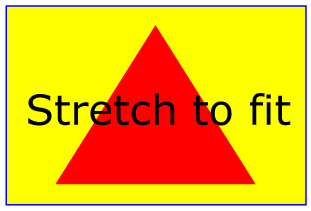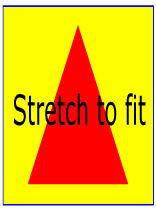The effect of the viewBox attribute is that the user agent automatically supplies the appropriate transformation matrix to map the specified rectangle in user space to the bounds of the viewport. To achieve the effect of the example on the left, with viewport dimensions of 300 by 200 pixels, the user agent needs to automatically insert a transformation which scales both X and Y by 0.2. The effect is equivalent to having a viewport of size 300px by 200px and the following supplemental transformation in the document, as follows:

```<?xml version="1.0" standalone="no"?>
<!DOCTYPE svg PUBLIC "-//W3C//DTD SVG 20000303 Stylable//EN"
"http://www.w3.org/TR/2000/03/WD-SVG-20000303/DTD/svg-20000303-stylable.dtd">
<svg width="300px" height="200px">

<g transform="scale(0.2)">

<!-- Rest of document goes here -->

</g>
</svg>
```
To achieve the effect of the example on the right, with viewport dimensions of 150 by 200 pixels, the user agent needs to automatically insert a transformation which scales X by 0.1 and Y by 0.2. The effect is equivalent to having a viewport of size 150px by 200px and the following supplemental transformation in the document, as follows:
```<?xml version="1.0" standalone="no"?>
<!DOCTYPE svg PUBLIC "-//W3C//DTD SVG 20000303 Stylable//EN"
"http://www.w3.org/TR/2000/03/WD-SVG-20000303/DTD/svg-20000303-stylable.dtd">
<svg width="150px" height="200px">

<g transform="scale(0.1 0.2)">

<!-- Rest of document goes here -->

</g>
</svg>
```

(Note: in some cases the user agent will need to supply a translate transformation in addition to a scale transformation. For example, on an outermost 'svg', a translate transformation will be needed if the viewBox attributes specifies values other than zero for <min-x> or <min-y>.)

For the viewBox attribute:

Animatable: yes.

## 7.8 The preserveAspectRatio attribute

In some cases when using the viewBox attribute, it is desirable that the graphics stretch to fit non-uniformly to take up the entire viewport. In other cases when using the viewBox attribute, it is desirable that uniform scaling be used for the purposes of preserving the aspect ratio of the graphics. Attribute preserveAspectRatio="<align> [<meetOrSlice>]", which is available for all elements that establish a new viewport (see elements that establish viewports), indicates whether or not to force uniform scaling. The <align> parameter indicates whether to force uniform scaling and, if so, the alignment method to use in case the aspect ratio of the viewBox doesn't match the aspect ratio of the viewport. The <align> parameter must be one of the following strings:

• none - Do not force uniform scaling. Scale the graphic content of the given element non-uniformly if necessary such that the element's bounding box exactly matches the viewport rectangle.
• xMinYMin - Force uniform scaling.
Align the <min-x> of the element's viewBox with the smallest X value of the viewport.
Align the <min-y> of the element's viewBox with the smallest Y value of the viewport.
• xMidYMin - Force uniform scaling.
Align the midpoint X value of the element's viewBox with the midpoint X value of the viewport.
Align the <min-y> of the element's viewBox with the smallest Y value of the viewport.
• xMaxYMin - Force uniform scaling.
Align the <min-x>+<width> of the element's viewBox with the maximum X value of the viewport.
Align the <min-y> of the element's viewBox with the smallest Y value of the viewport.
• xMinYMid - Force uniform scaling.
Align the <min-x> of the element's viewBox with the smallest X value of the viewport.
Align the midpoint Y value of the element's viewBox with the midpoint Y value of the viewport.
• xMidYMid (the default) - Force uniform scaling.
Align the midpoint X value of the element's viewBox with the midpoint X value of the viewport.
Align the midpoint Y value of the element's viewBox with the midpoint Y value of the viewport.
• xMaxYMid - Force uniform scaling.
Align the <min-x>+<width> of the element's viewBox with the maximum X value of the viewport.
Align the midpoint Y value of the element's viewBox with the midpoint Y value of the viewport.
• xMinYMax - Force uniform scaling.
Align the <min-x> of the element's viewBox with the smallest X value of the viewport.
Align the <min-y>+<height> of the element's viewBox with the maximum Y value of the viewport.
• xMidYMax - Force uniform scaling.
Align the midpoint X value of the element's viewBox with the midpoint X value of the viewport.
Align the <min-y>+<height> of the element's viewBox with the maximum Y value of the viewport.
• xMaxYMax - Force uniform scaling.
Align the <min-x>+<width> of the element's viewBox with the maximum X value of the viewport.
Align the <min-y>+<height> of the element's viewBox with the maximum Y value of the viewport.

The <meetOrSlice> parameter is optional and must be one of the following strings:

• meet (the default) - Scale the graphic such that:
• aspect ratio is preserved
• the entire viewBox is visible within the viewport
• the viewBox is scaled up as much as possible, while still meeting the other criteria
In this case, if the aspect ratio of the graphic does not match the viewport, some of the viewport will extend beyond the bounds of the viewBox (i.e., the area into which the viewBox will draw will be smaller than the viewport).
• slice - Scale the graphic such that:
• aspect ratio is preserved
• the entire viewport is covered by the viewBox
• the viewBox is scaled down as much as possible, while still meeting the other criteria
In this case, if the aspect ratio of the viewBox does not match the viewport, some of the viewBox will extend beyond the bounds of the viewport (i.e., the area into which the viewBox will draw is larger than the viewport).

Example PreserveAspectRatio illustrates the various options on preserveAspectRatio. To save space, XML entities have been defined for the three repeated graphic objects, the rectangle with the smile inside and the outlines of the two rectangles which have the same dimensions as the target viewports. The example creates several new viewports by including 'svg' sub-elements embedded inside the outermost 'svg' element (see Establishing a new viewport). The smile is drawing the text string ":)" rotated 90 degrees.

```<?xml version="1.0" standalone="no"?>
<!DOCTYPE svg PUBLIC "-//W3C//DTD SVG 20000303 Stylable//EN"
"http://www.w3.org/TR/2000/03/WD-SVG-20000303/DTD/svg-20000303-stylable.dtd" [
<!ENTITY Smile "
<rect x='.5' y='.5' width='29' height='39' style='fill:yellow; stroke:red'/>
<g transform='rotate(90)'>
<text x='10' y='10' style='font-family:Verdana;
font-weight:bold; font-size:14'>:)</text>
</g>">
<!ENTITY Viewport1 "<rect x='.5' y='.5' width='49' height='29'
style='fill:none; stroke:blue'/>">
<!ENTITY Viewport2 "<rect x='.5' y='.5' width='29' height='59'
style='fill:none; stroke:blue'/>">
]>
<svg width="480px" height="270px" style="font-family:Verdana; font-size:8">
<desc>Example PreserveAspectRatio - demonstrate available options</desc>
<text x="10" y="30">SVG to fit</text>
<g transform="translate(20,40)">&Smile;</g>
<text x="10" y="110">Viewport 1</text>
<g transform="translate(10,120)">&Viewport1;</g>
<text x="10" y="180">Viewport 2</text>
<g transform="translate(20,190)">&Viewport2;</g>
<text x="100" y="30">--------------- meet ---------------</text>
<g transform="translate(100,60)"><text y="-10">xMin*</text>&Viewport1;
<svg preserveAspectRatio="xMinYMin meet" viewBox="0 0 30 40"
width="50" height="30">&Smile;</svg></g>
<g transform="translate(170,60)"><text y="-10">xMid*</text>&Viewport1;
<svg preserveAspectRatio="xMidYMid meet" viewBox="0 0 30 40"
width="50" height="30">&Smile;</svg></g>
<g transform="translate(240,60)"><text y="-10">xMax*</text>&Viewport1;
<svg preserveAspectRatio="xMaxYMax meet" viewBox="0 0 30 40"
width="50" height="30">&Smile;</svg></g>
<text x="330" y="30">---------- meet ----------</text>
<g transform="translate(330,60)"><text y="-10">*YMin</text>&Viewport2;
<svg preserveAspectRatio="xMinYMin meet" viewBox="0 0 30 40"
width="30" height="60">&Smile;</svg></g>
<g transform="translate(380,60)"><text y="-10">*YMid</text>&Viewport2;
<svg preserveAspectRatio="xMidYMid meet" viewBox="0 0 30 40"
width="30" height="60">&Smile;</svg></g>
<g transform="translate(430,60)"><text y="-10">*YMax</text>&Viewport2;
<svg preserveAspectRatio="xMaxYMax meet" viewBox="0 0 30 40"
width="30" height="60">&Smile;</svg></g>
<text x="100" y="160">---------- slice ----------</text>
<g transform="translate(100,190)"><text y="-10">xMin*</text>&Viewport2;
<svg preserveAspectRatio="xMinYMin slice" viewBox="0 0 30 40"
width="30" height="60">&Smile;</svg></g>
<g transform="translate(150,190)"><text y="-10">xMid*</text>&Viewport2;
<svg preserveAspectRatio="xMidYMid slice" viewBox="0 0 30 40"
width="30" height="60">&Smile;</svg></g>
<g transform="translate(200,190)"><text y="-10">xMax*</text>&Viewport2;
<svg preserveAspectRatio="xMaxYMax slice" viewBox="0 0 30 40"
width="30" height="60">&Smile;</svg></g>
<text x="270" y="160">--------------- slice ---------------</text>
<g transform="translate(270,190)"><text y="-10">*YMin</text>&Viewport1;
<svg preserveAspectRatio="xMinYMin slice" viewBox="0 0 30 40"
width="50" height="30">&Smile;</svg></g>
<g transform="translate(340,190)"><text y="-10">*YMid</text>&Viewport1;
<svg preserveAspectRatio="xMidYMid slice" viewBox="0 0 30 40"
width="50" height="30">&Smile;</svg></g>
<g transform="translate(410,190)"><text y="-10">*YMax</text>&Viewport1;
<svg preserveAspectRatio="xMaxYMax slice" viewBox="0 0 30 40"
width="50" height="30">&Smile;</svg></g>
</svg>
```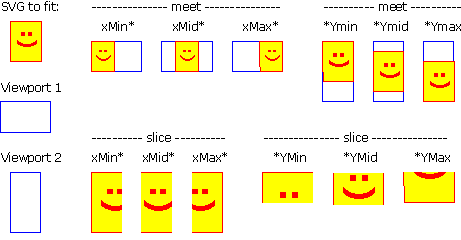For the preserveAspectRatio attribute:

Animatable: yes.

## 7.9 Establishing a new viewport

At any point in an SVG drawing, you can establish a new viewport into which all contained graphics is drawn by including an 'svg' element inside SVG content. By establishing a new viewport, you also implicitly establish a new initial user space, new meanings for many of the CSS unit specifiers and, potentially, a new clipping path. The bounds of the new viewport are defined by the x, y, width and height attributes on the 'svg' element. Here is an example:

```<?xml version="1.0" standalone="no"?>
<!DOCTYPE svg PUBLIC "-//W3C//DTD SVG 20000303 Stylable//EN"
"http://www.w3.org/TR/2000/03/WD-SVG-20000303/DTD/svg-20000303-stylable.dtd">
<svg width="4in" height="3in">
<desc>This SVG drawing embeds another one,
thus establishing a new viewport
</desc>
<!-- The following statement establishing a new viewport
and renders SVG drawing B into that viewport -->
<svg x="25%" y="25%" width="50%" height="50%">
<!-- drawing B goes here -->
</svg>
</svg>
```

For an extensive example of creating new viewports, see Example PreserveAspectRatio.

In addition to the 'svg' element, the following other elements also establish a new viewport:

• A 'use' or 'image' element establishes a temporary new viewport for drawing instances of referenced elements or files
• A 'marker' element establishes a temporary new viewport for drawing arrowheads and polymarkers
• When a pattern is used to fill or stroke an object by reference to a 'pattern' element, a temporary new viewport is established for each drawn instance of the pattern.
• When a 'mask' element is used to establish a mask for an object and maskUnits="objectBoundingBox", a temporary new viewport is established to draw the elements within the 'mask' element.
• A 'foreignObject' element creates a new viewport for rendering the content that is within the element.

Whether a new viewport also establishes a new additional clipping path is determined by the value of the 'overflow' property on the element which establishes the new viewport. If a clipping path is created to correspond to the new viewport, the clipping path's geometry is determined by the value of the 'clip' property. Also, see Clip to viewport vs. clip to viewBox.

## 7.10 Units

All coordinates and lengths in SVG can be specified in one of the following ways:

• User units. If no unit specifier is provided, a given coordinate or length is assumed to be in user units (i.e., a value in user space). For example:
```<text style="font-size: 50">Text size is 50 user units</text>
```
• CSS units. If a CSS unit specifier is provided on a coordinate or length value, then the given value is assumed to be in CSS units. Available CSS unit specifiers are the absolute and relative unit specifiers from CSS (em, ex, px, pt, pc, cm, mm, in and percentages). As in CSS, the em and ex unit specifiers are relative to the current font's font-size and x-height, respectively. Initially, the various absolute unit specifiers from CSS (i.e., px, pt, pc, cm, mm, in) represent lengths within the initial user coordinate system and do not change their meaning as transformations alter the current coordinate system. Thus, "12pt" can be made to represent exactly 12 points on the actual visual medium even if the user coordinate system has been scaled. For example:
```<?xml version="1.0" standalone="no"?>
<!DOCTYPE svg PUBLIC "-//W3C//DTD SVG 20000303 Stylable//EN"
"http://www.w3.org/TR/2000/03/WD-SVG-20000303/DTD/svg-20000303-stylable.dtd">
<svg width="4in" height="3in">
<desc>Demonstration of coordinate transforms
</desc>
<!-- The following two text elements will both draw with a
font height of 12 pixels -->
<text style="font-size: 12">This prints 12 pixels high.</text>
<text style="font-size: 12px">This prints 12 pixels high.</text>

<!-- Now scale the coordinate system by 2. -->
<g transform="scale(2)">

<!-- The following text will actually draw 24 pixels high
because each unit in the new coordinate system equals
2 units in the previous coordinate system. -->
<text style="font-size: 12">This prints 24 pixels high.</text>

<!-- The following text will actually still draw 12 pixels high
because the CSS unit specifier has been provided. -->
<text style="font-size: 12px">This prints 12 pixels high.</text>

</g>
</svg>
```

Download this example

If possible, the SVG user agent must be passed the actual size of a px unit in inches or millimeters by its parent user agent. (See Conformance Requirements and Recommendations.) If such information is not available from the parent user agent, then the SVG user agent shall assume a px is defined to be exactly .28mm.

## 7.11 Redefining the meaning of CSS unit specifiers

The process of establishing a new viewport, such as when there is 'svg' element inside of another SVG 'svg', changes the meaning of the following CSS unit specifiers: px, pt, pc, cm, mm, in, and % (percentages). A "pixel" (the px unit) becomes equivalent to a single unit in the user coordinate system for the given 'svg' element. The meaning of the other absolute unit specifiers (pt, pc, cm, mm, in) are determined as an appropriate multiple of a px unit using the actual size of px unit (as passed from the parent user agent to the SVG user agent). Any percentage values that are relative to the current viewport will also represent new values.

```<?xml version="1.0" standalone="no"?>
<!DOCTYPE svg PUBLIC "-//W3C//DTD SVG 20000303 Stylable//EN"
"http://www.w3.org/TR/2000/03/WD-SVG-20000303/DTD/svg-20000303-stylable.dtd">
<svg width="300px" height="300px">
<desc>Transformation with establishment of a new viewport
</desc>
<!-- The following two text elements will both draw with a
font height of 12 pixels -->
<text style="font-size: 12">This prints 12 pixels high.</text>
<text style="font-size: 12px">This prints 12 pixels high.</text>

<!-- Now scale the coordinate system by 2. -->
<g transform="scale(2)">

<!-- The following text will actually draw 24 pixels high
because each unit in the new coordinate system equals
2 units in the previous coordinate system. -->
<text style="font-size: 12">This prints 24 pixels high.</text>

<!-- The following text will actually still draw 12 pixels high
because the CSS unit specifier has been provided. -->
<text style="font-size: 12px">This prints 12 pixels high.</text>
</g>

<!-- This time, scale the coordinate system by 3. -->
<g transform="scale(3)">

<!-- Establish a new viewport and thus change the meaning of
some CSS unit specifiers. -->
<svg style="left:0; top:0; right:100; bottom:100"
width="100%" height="100%">

<!-- The following two text elements will both draw with a
font height of 36 screen pixels. The first text element
defines its height in user coordinates, which have been
scaled by 3. The second text element defines its height
in CSS px units, which have been redefined to be three times
as big as screen pixels due the <svg> element establishing
a new viewport. -->
<text style="font-size: 12">This prints 36 pixels high.</text>
<text style="font-size: 12px">This prints 36 pixels high.</text>

</svg>
</g>
</svg>
```

Download this example

## 7.12 Processing rules for CSS units and percentages

Any values expressed in CSS units or percentages of the current viewport are mapped to corresponding values in user space as follows:

• For any x-coordinate value or width value (xValueInVPSpace) expressed using CSS units (other than percentages), first convert xValueInVPSpace into viewport pixel units using the SVG user agent's standard conversion factor from pixels to real world units (e.g., millimeters) to yield xValueInVPPixels. Then transform the points (0,0) and (xValueInVPPixels,0), from viewport space to current user space using the inverse of the current transformation matrix, yielding two points in userspace Q1 and Q2. Do a distance calculation between Q1 and Q2 (sqrt((Q2x-Q1x)**2 + (Q2y-Q1y)**2)) and use that as the value for the given operation.
• For any y-coordinate value or height value (yValueInVPSpace) expressed using CSS units (other than percentages), then use the same method as above, except use points (0,0) and (0,yValueInVPPixels) instead.
• For any x-coordinate value or width value (xValueInVPSpace) expressed as a percentage of the viewport, transform the points (0,0) and (percentageValue*vpWidthInPixels,0), from viewport space to current user space using the inverse of the current transformation matrix, yielding two points in userspace Q1 and Q2. Do a distance calculation between Q1 and Q2 (sqrt((Q2x-Q1x)**2 + (Q2y-Q1y)**2)) and use that as the value for the given operation.
• For any y-coordinate value or height value (yValueInVPSpace) expressed as a percentage of the viewport, then use the same method as above, except use points (0,0) and (0,percentageValue*vpHeightInPixels) instead.
• For any other length value in viewport space (lengthVPSpace), the following approach is used to give appropriate weighting to the contribution of the two dimensions of the viewport. First, convert lengthVPSpace into viewport pixel units using the SVG user agent's standard conversion factor from pixels to to real world units (e.g., millimeters) to yield lengthVPPixels. Calculate the distance from (0,0) and (vpWidthInPixels,vpHeightInPixels) in viewport space using the formula: vpDiagLengthVPPixels=sqrt(vpWidthInPixels**2 + vpHeightInPixels**2). Using the inverse of the current transformation matrix, determine the points in user space (P1x,P1y) and (P2x,P2y) which correspond to the points (0,0) and (vpWidthInPixels,vpHeightInPixels) in viewport space. Calculate the distance from (P1x,P1y) and (P2x,P2y) in user space using the formula: vpDiagLengthUserSpace=sqrt((P2x-P1x)**2 + (P2y-P1y)**2)). Then, convert the original viewport-relative length into a length in user space using the formula: lengthUserSpace = lengthVPPixels * (vpDiagLengthUserSpace/vpDiagLengthVPPixels).
• If a viewport-relative percentage value is given, then use the same method as above, except calculate lengthVPPixels as lengthVPPixels=percentageValue*sqrt(vpWidthPixels**2 + vpHeightPixels**2)/sqrt(2).

Any values expressed as fractions or percentages of the current object bounding box are mapped to corresponding values in user space as follows:

• For any x-coordinate value or width value, determine the minimum and maximum x-coordinates in user space for the object bounding box (bboxXMinUserSpace and bboxXMaxUserSpace, respectively). An x-coordinate value is converted into a coordinate in user space using the formula bboxXMinUserSpace+percentageValue*(bboxXMaxUserSpace-bboxXMinUserSpace) and a width value is converted into a length in user space using the formula percentageValue*(bboxXMaxUserSpace-bboxXMinUserSpace).
• For any y-coordinate value or width value, determine the minimum and maximum y-coordinates in user space for the object bounding box (bboxYMinUserSpace and bboxYMaxUserSpace, respectively). A y-coordinate value is converted into a coordinate in user space using the formula bboxYMinUserSpace+percentageValue*(bboxYMaxUserSpace-bboxYMinUserSpace) and a height value is converted into a length in user space using the formula percentageValue*(bboxYMaxUserSpace-bboxYMinUserSpace).
• For any other length value expressed as a fraction or percentage of the current object bounding box, determine the minimum and maximum x and y coordinates in user space for the object bounding box (bboxXMinUserSpace, bboxXMaxUserSpace, bboxYMinUserSpace and bboxYMaxUserSpace), calculate bboxWidth=bboxXMaxUserSpace-bboxXMinUserSpace and bboxHeight=bboxYMaxUserSpace-bboxYMinUserSpace, and then map the fraction or percentage of the current object bounding box to a length in user space using the formula percentageValue*sqrt(bboxWidth**2 + bboxHeight**2)/sqrt(2).

## 7.13 DOM interfaces

The following interfaces are defined below: SVGPoint, SVGMatrix, SVGTransformList, SVGTransform, SVGPreserveAspectRatio, SVGFitToViewBox, SVGTransformable.

### Interface SVGPoint

Many of the SVG DOM interfaces refer to objects of class SVGPoint. An SVGPoint is an (x,y) coordinate pair. When used in matrix operations, an SVGPoint is treated as a vector of the form:

```[x]
[y]
```

IDL Definition
```interface SVGPoint {
attribute SVGLength x;
attribute SVGLength y;

SVGPoint matrixTransform ( in SVGMatrix matrix )
raises( SVGException );
};
```

Attributes
SVGLength x
The x coordinate.
SVGLength y
The y coordinate.
Methods
matrixTransform

Applies a 2x3 matrix transformation on this SVGPoint object and returns a new, transformed SVGPoint object:

`newpoint = matrix * thispoint`
Parameters
 in SVGMatrix matrix The matrix which is to be applied to this SVGPoint object.
Return value
 SVGPoint A new SVGPoint object.
Exceptions
 SVGException SVG_WRONG_TYPE_ERR: Raised if parameter matrix is of the wrong type.

### Interface SVGMatrix

Many of SVG's graphics operations utilize 2x3 matrices of the form:

```[a c e]
[b d f]```

which, when expanded into a 3x3 matrix for the purposes of matrix arithmetic, become:

```[a c e]
[b d f]
[0 0 1]```

IDL Definition
```interface SVGMatrix {
attribute float      a;
attribute float      b;
attribute float      c;
attribute float      d;
attribute SVGLength e;
attribute SVGLength f;

SVGMatrix multiply ( in SVGMatrix secondMatrix )
raises( SVGException );
SVGMatrix inverse (  )
raises( SVGException );
SVGMatrix translate ( in SVGLength x, in SVGLength y )
raises( SVGException );
SVGMatrix scale ( in float scaleFactor )
raises( SVGException );
SVGMatrix scaleNonUniform ( in float scaleFactorX, in float scaleFactorY )
raises( SVGException );
SVGMatrix rotate ( in SVGAngle angle )
raises( SVGException );
SVGMatrix rotateFromVector ( in SVGLength x, in SVGLength y )
raises( SVGException );
SVGMatrix flipX (  );
SVGMatrix flipY (  );
SVGMatrix skewX ( in SVGAngle angle )
raises( SVGException );
SVGMatrix skewY ( in SVGAngle angle )
raises( SVGException );
};
```

Attributes
float a
The a component of the matrix.
float b
The b component of the matrix.
float c
The c component of the matrix.
float d
The d component of the matrix.
SVGLength e
The e component of the matrix.
SVGLength f
The f component of the matrix.
Methods
multiply
Performs matrix multiplication. This matrix is post-multiplied by another matrix, returning the resulting new matrix.
Parameters
 in SVGMatrix secondMatrix The matrix which is post-multiplied to this matrix.
Return value
 SVGMatrix The resulting matrix.
Exceptions
 SVGException SVG_WRONG_TYPE_ERR: Raised if parameter secondMatrix is of the wrong type.
inverse
Returns the inverse matrix.
No Parameters
Return value
 SVGMatrix The inverse matrix.
Exceptions
 SVGException SVG_MATRIX_NOT_INVERTABLE: Raised if this matrix is not invertable.
translate
Post-multiplies a translation transformation on the current matrix and returns the resulting matrix.
Parameters
 in SVGLength x The distance to translate along the X axis. in SVGLength y The distance to translate along the Y axis.
Return value
 SVGMatrix The resulting matrix.
Exceptions
 SVGException SVG_WRONG_TYPE_ERR: Raised if one of the parameters is of the wrong type.
scale
Post-multiplies a uniform scale transformation on the current matrix and returns the resulting matrix.
Parameters
 in float scaleFactor Scale factor in both X and Y.
Return value
 SVGMatrix The resulting matrix.
Exceptions
 SVGException SVG_WRONG_TYPE_ERR: Raised if one of the parameters is of the wrong type.
scaleNonUniform
Post-multiplies a non-uniform scale transformation on the current matrix and returns the resulting matrix.
Parameters
 in float scaleFactorX Scale factor in X. in float scaleFactorY Scale factor in Y.
Return value
 SVGMatrix The resulting matrix.
Exceptions
 SVGException SVG_WRONG_TYPE_ERR: Raised if one of the parameters is of the wrong type.
rotate
Post-multiplies a rotation transformation on the current matrix and returns the resulting matrix.
Parameters
 in SVGAngle angle Rotation angle.
Return value
 SVGMatrix The resulting matrix.
Exceptions
 SVGException SVG_WRONG_TYPE_ERR: Raised if one of the parameters is of the wrong type.
rotateFromVector
Post-multiplies a rotation transformation on the current matrix and returns the resulting matrix. The rotation angle is determined by taking (+/-) atan(y/x). The direction of the vector (x,y) determines whether the positive or negative angle value is used.
Parameters
 in SVGLength x The X coordinate of the vector (x,y). Must not be zero. in SVGLength y The Y coordinate of the vector (x,y). Must not be zero.
Return value
 SVGMatrix The resulting matrix.
Exceptions
 SVGException SVG_WRONG_TYPE_ERR: Raised if one of the parameters is of the wrong type. SVG_INVALID_VALUE_ERR: Raised if one of the parameters has an invalid value.
flipX
Post-multiplies the transformation [-1 0 0 1 0 0] and returns the resulting matrix.
No Parameters
Return value
 SVGMatrix The resulting matrix.
No Exceptions
flipY
Post-multiplies the transformation [1 0 0 -1 0 0] and returns the resulting matrix.
No Parameters
Return value
 SVGMatrix The resulting matrix.
No Exceptions
skewX
Post-multiplies a skewX transformation on the current matrix and returns the resulting matrix.
Parameters
 in SVGAngle angle Skew angle.
Return value
 SVGMatrix The resulting matrix.
Exceptions
 SVGException SVG_WRONG_TYPE_ERR: Raised if one of the parameters is of the wrong type.
skewY
Post-multiplies a skewY transformation on the current matrix and returns the resulting matrix.
Parameters
 in SVGAngle angle Skew angle.
Return value
 SVGMatrix The resulting matrix.
Exceptions
 SVGException SVG_WRONG_TYPE_ERR: Raised if one of the parameters is of the wrong type.

### Interface SVGTransformList

SVGTransformList maintains an ordered list of SVGTransform objects. The SVGTransformList and SVGTransform interfaces correspond to the various attributes which specify a set of transformations, such as the transform attribute which is available for many of SVG's elements.

The various methods inherited from SVGList, which are defined in SVGList to accept parameters and return values of type Object, must receive parameters of type SVGTransform and return values of type SVGTransform.

IDL Definition
```interface SVGTransformList : SVGList {
SVGTransform createSVGTransformFromMatrix ( in SVGMatrix matrix )
raises( SVGException );
SVGTransform consolidate (  );
};
```

Methods
createSVGTransformFromMatrix
Creates an SVGTransform object which is initialized to transform of type SVG_TRANSFORM_MATRIX and whose values are the given matrix.
Parameters
 in SVGMatrix matrix The matrix which defines the transformation.
Return value
 SVGTransform The returned SVGTransform object.
Exceptions
 SVGException SVG_WRONG_TYPE_ERR: Raised if parameter newItem is the wrong type of object for the given list.
consolidate
Consolidates the list of separate SVGTransform objects by multiplying the equivalent transformation matrices together to result in a list consisting of a single SVGTransform object of type SVG_TRANSFORM_MATRIX.
No Parameters
Return value
 SVGTransform The resulting SVGTransform object which becomes single item in the list. If the list was empty, then a value of null is returned.
No Exceptions

### Interface SVGTransform

SVGTransform is the interface for one of the component transformations within a SVGTransformList; thus, a SVGTransform object corresponds to single component (e.g., "scale(..)" or "matrix(...)") within a transform attribute specification.

IDL Definition
```interface SVGTransform {
// Transform Types
constant unsigned short SVG_TRANSFORM_UNKNOWN   = 0;
constant unsigned short SVG_TRANSFORM_MATRIX    = 1;
constant unsigned short SVG_TRANSFORM_TRANSLATE = 2;
constant unsigned short SVG_TRANSFORM_SCALE     = 3;
constant unsigned short SVG_TRANSFORM_ROTATE    = 4;
constant unsigned short SVG_TRANSFORM_SKEWX     = 5;
constant unsigned short SVG_TRANSFORM_SKEWY     = 6;

readonly attribute unsigned short type;
readonly attribute SVGMatrix matrix;
readonly attribute SVGAngle angle;

void setMatrix ( in SVGMatrix matrix )
raises( SVGException );
void setTranslate ( in SVGLength tx, in SVGLength ty )
raises( SVGException );
void setScale ( in SVGNumber sx, in SVGNumber sy )
raises( SVGException );
void setRotate ( in SVGAngle angle )
raises( SVGException );
void setSkewX ( in SVGAngle angle )
raises( SVGException );
void setSkewY ( in SVGAngle angle )
raises( SVGException );
};
```

Definition group Transform Types
Defined constants
 SVG_TRANSFORM_UNKNOWN The unit type is not one of predefined types. It is invalid to attempt to define a new value of this type or to attempt to switch an existing value to this type. SVG_TRANSFORM_MATRIX A "matrix(...)" transformation. SVG_TRANSFORM_TRANSLATE A "translate(...)" transformation. SVG_TRANSFORM_SCALE A "scale(...)" transformation. SVG_TRANSFORM_ROTATE A "rotate(...)" transformation. SVG_TRANSFORM_SKEWX A "skewX(...)" transformation. SVG_TRANSFORM_SKEWY A "skewY(...)" transformation.
Attributes
readonly unsigned short type
The type of the value as specified by one of the constants specified above.
readonly SVGMatrix matrix
The matrix that represents this transformation.
For SVG_TRANSFORM_MATRIX, the matrix contains the a, b, c, d, e, f values supplied by the user.
For SVG_TRANSFORM_TRANSLATE, e and f represents the translation amounts (a=1,b=0,c=0,d=1).
For SVG_TRANSFORM_SCALE, a and d represents the scale amounts (b=0,c=0,e=0,f=0).
For SVG_TRANSFORM_ROTATE, SVG_TRANSFORM_SKEWX and SVG_TRANSFORM_SKEWY, a, b, c and d represent the matrix which will result in the given transformation (e=0,f=0).
readonly SVGAngle angle
A convenience attribute for SVG_TRANSFORM_ROTATE, SVG_TRANSFORM_SKEWX and SVG_TRANSFORM_SKEWY. It holds the angle that was specified.
For SVG_TRANSFORM_MATRIX, SVG_TRANSFORM_TRANSLATE and SVG_TRANSFORM_SCALE, angle will be zero.
Methods
setMatrix
Sets the transform type to SVG_TRANSFORM_MATRIX, with parameter matrix defining the new transformation.
Parameters
 in SVGMatrix matrix The new matrix for the transformation.
No Return Value
Exceptions
 SVGException SVG_WRONG_TYPE_ERR: Raised if the parameter is of the wrong type.
setTranslate
Sets the transform type to SVG_TRANSFORM_TRANSLATE, with parameters tx and ty defining the translation amounts.
Parameters
 in SVGLength tx The translation amount in X. in SVGLength ty The translation amount in Y.
No Return Value
Exceptions
 SVGException SVG_WRONG_TYPE_ERR: Raised if the parameter is of the wrong type.
setScale
Sets the transform type to SVG_TRANSFORM_SCALE, with parameters sx and sy defining the scale amounts.
Parameters
 in SVGNumber sx The scale factor in X. in SVGNumber sy The scale factor in Y.
No Return Value
Exceptions
 SVGException SVG_WRONG_TYPE_ERR: Raised if the parameter is of the wrong type.
setRotate
Sets the transform type to SVG_TRANSFORM_ROTATE, with parameter angle defining the rotation angle.
Parameters
 in SVGAngle angle The rotation angle.
No Return Value
Exceptions
 SVGException SVG_WRONG_TYPE_ERR: Raised if the parameter is of the wrong type.
setSkewX
Sets the transform type to SVG_TRANSFORM_SKEWX, with parameter angle defining the amount of skew.
Parameters
 in SVGAngle angle The skew angle.
No Return Value
Exceptions
 SVGException SVG_WRONG_TYPE_ERR: Raised if the parameter is of the wrong type.
setSkewY
Sets the transform type to SVG_TRANSFORM_SKEWY, with parameter angle defining the amount of skew.
Parameters
 in SVGAngle angle The skew angle.
No Return Value
Exceptions
 SVGException SVG_WRONG_TYPE_ERR: Raised if the parameter is of the wrong type.

### Interface SVGPreserveAspectRatio

The SVGPreserveAspectRatio interface corresponds to the preserveAspectRatio attribute, which is available for some of SVG's elements.

IDL Definition
```interface SVGPreserveAspectRatio {
// Alignment Types
constant unsigned short SVG_PRESERVEASPECTRATIO_NONE     = 0;
constant unsigned short SVG_PRESERVEASPECTRATIO_XMINYMIN = 1;
constant unsigned short SVG_PRESERVEASPECTRATIO_XMIDYMIN = 2;
constant unsigned short SVG_PRESERVEASPECTRATIO_XMAXYMIN = 3;
constant unsigned short SVG_PRESERVEASPECTRATIO_XMINYMID = 4;
constant unsigned short SVG_PRESERVEASPECTRATIO_XMIDYMID = 5;
constant unsigned short SVG_PRESERVEASPECTRATIO_XMAXYMID = 6;
constant unsigned short SVG_PRESERVEASPECTRATIO_XMINYMAX = 7;
constant unsigned short SVG_PRESERVEASPECTRATIO_XMIDYMAX = 8;
constant unsigned short SVG_PRESERVEASPECTRATIO_XMAXYMAX = 9;
// Meet-or-slice Types
constant unsigned short SVG_MEETORSLICE_MEET  = 0;
constant unsigned short SVG_MEETORSLICE_SLICE = 1;

attribute unsigned short align;
attribute unsigned short meetOrSlice;
};
```

Definition group Alignment Types
Defined constants
 SVG_PRESERVEASPECTRATIO_NONE Corresponds to value 'none' for attribute preserveAspectRatio. SVG_PRESERVEASPECTRATIO_XMINYMIN Corresponds to value 'xMinYMin' for attribute preserveAspectRatio. SVG_PRESERVEASPECTRATIO_XMIDYMIN Corresponds to value 'xMidYMin' for attribute preserveAspectRatio. SVG_PRESERVEASPECTRATIO_XMAXYMIN Corresponds to value 'xMaxYMin' for attribute preserveAspectRatio. SVG_PRESERVEASPECTRATIO_XMINYMID Corresponds to value 'xMinYMid' for attribute preserveAspectRatio. SVG_PRESERVEASPECTRATIO_XMIDYMID Corresponds to value 'xMidYMid' for attribute preserveAspectRatio. SVG_PRESERVEASPECTRATIO_XMAXYMID Corresponds to value 'xMaxYMid' for attribute preserveAspectRatio. SVG_PRESERVEASPECTRATIO_XMINYMAX Corresponds to value 'xMinYMax' for attribute preserveAspectRatio. SVG_PRESERVEASPECTRATIO_XMIDYMAX Corresponds to value 'xMidYMax' for attribute preserveAspectRatio. SVG_PRESERVEASPECTRATIO_XMAXYMAX Corresponds to value 'xMaxYMax' for attribute preserveAspectRatio.
Definition group Meet-or-slice Types
Defined constants
 SVG_MEETORSLICE_MEET Corresponds to value 'meet' for attribute preserveAspectRatio. SVG_MEETORSLICE_SLICE Corresponds to value 'slice' for attribute preserveAspectRatio.
Attributes
unsigned short align
The type of the alignment value as specified by one of the constants specified above.
unsigned short meetOrSlice
The type of the meet-or-slice value as specified by one of the constants specified above.

### Interface SVGFitToViewBox

Interface SVGFitToViewBox defines DOM attributes that apply to elements which have XML attributes viewBox and preserveAspectRatio.

IDL Definition
```interface SVGFitToViewBox {
attribute SVGRect viewBox;
attribute SVGPreserveAspectRatio preserveAspectRatio;
};
```

Attributes
SVGRect viewBox
Corresponds to attribute viewBox on the given element.
SVGPreserveAspectRatio preserveAspectRatio
Corresponds to attribute preserveAspectRatio on the given element.

### Interface SVGTransformable

Interface SVGTransformable contains properties and methods that apply to all elements which have attribute transform.

IDL Definition
```interface SVGTransformable {
readonly attribute SVGElement       nearestViewportElement;
readonly attribute SVGElement       farthestViewportElement;
attribute SVGTransformList transform;

SVGRect   getBBox (  );
SVGMatrix getCTM (  );
SVGMatrix getScreenCTM (  );
SVGMatrix getTransformToElement ( in SVGElement element )
raises( SVGException );
};
```

Attributes
readonly SVGElement nearestViewportElement
The element which established the current viewport. Often, the nearest ancestor 'svg' element. Null if this is the given element is the outermost 'svg' element.
readonly SVGElement farthestViewportElement
The farthest ancestor 'svg' element. Null if this is the given element is the outermost 'svg' element.
SVGTransformList transform
Corresponds to attribute transform on the given element.
Methods
getBBox
Returns the tight bounding box in current user space (i.e., after application of the transform attribute) on the geometry of all contained graphics elements, exclusive of stroke-width and filter effects.
No Parameters
Return value
 SVGRect An SVGRect object that defines the bounding box.
No Exceptions
getCTM
Returns the transformation matrix from current user units (i.e., after application of the transform attribute) to the viewport coordinate system for the nearestViewportElement.
No Parameters
Return value
 SVGMatrix An SVGMatrix object that defines the CTM.
No Exceptions
getScreenCTM
Returns the transformation matrix from current user units (i.e., after application of the transform attribute) to the parent user agent's notice of a "pixel". For display devices, ideally this represents a physical screen pixel. For other devices or environments where physical pixel sizes are not know, then an algorithm similar to the CSS2 definition of a "pixel" can be used instead.
No Parameters
Return value
 SVGMatrix An SVGMatrix object that defines the given transformation matrix.
No Exceptions
getTransformToElement
Returns the transformation matrix from the user coordinate system on the current element (after application of the transform attribute) to the user coordinate system on element (after application of its transform attribute).
Parameters
 in SVGElement element The target element.
Return value
 SVGMatrix An SVGMatrix object that defines the transformation.
Exceptions
 SVGException SVG_WRONG_TYPE_ERR: Raised if the target element is of an invalid type. SVG_MATRIX_NOT_INVERTABLE: Raised if the currently defined transformation matrices make it impossible to compute the given matrix (e.g., because one of the transformations is singular).